Courses

# Common Base Amplifier Electrical Engineering (EE) Notes | EduRev

## Electronic Devices

Created by: Cstoppers Instructors

## Electrical Engineering (EE) : Common Base Amplifier Electrical Engineering (EE) Notes | EduRev

The document Common Base Amplifier Electrical Engineering (EE) Notes | EduRev is a part of the Electrical Engineering (EE) Course Electronic Devices.
All you need of Electrical Engineering (EE) at this link: Electrical Engineering (EE)

Common Base Amplifier:

 The common base amplifier circuit is shown in Fig. 1. The VEE source forward biases the emitter diode and VCC source reverse biases collector diode. The ac source Vin is connected to emitter through a coupling capacitor so that it blocks dc. This ac voltage produces small fluctuations in currents and voltages. The load resistance RL is also connected to collector through coupling capacitor so the fluctuations in collector base voltage will be observed across RL.The dc equivalent circuit is obtained by reducing all ac sources to zero and opening all capacitors. The dc collector current is same as IE and VCB is given byVCB = VCC - IC RC.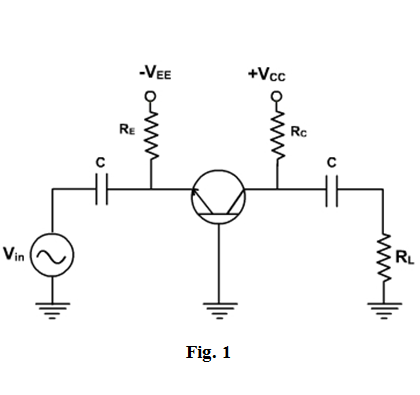These current and voltage fix the Q point. The ac equivalent circuit is obtained by reducing all dc sources to zero and shorting all coupling capacitors. r'e represents the ac resistance of the diode as shown in Fig. 2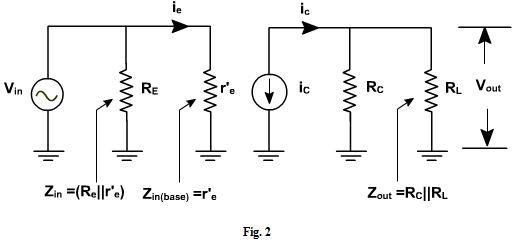Fig. 3, shows the diode curve relating IE and VBE. In the absence of ac signal, the transistor operates at Q point (point of intersection of load line and input characteristic). When the ac signal is applied, the emitter current and voltage also change.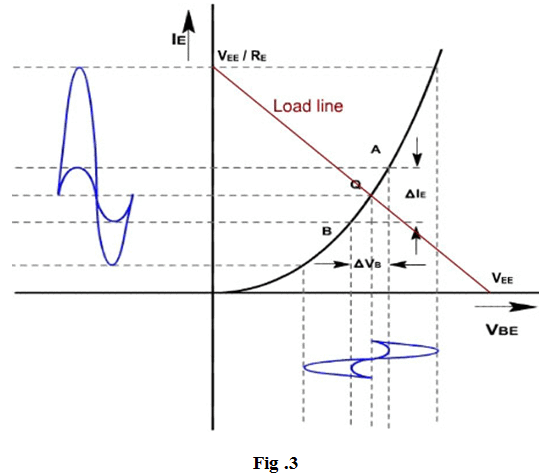If the ac signal is small, the points A and B are close to Q, and arc A B can be approximated by a straight line and diode appears to be a resistance given by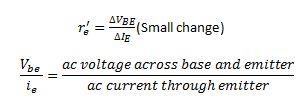If the input signal is small, input voltage and current will be sinusoidal but if the input voltage is large then current will no longer be sinusoidal because of the non linearity of diode curve. The emitter current is elongated on the positive half cycle and compressed on negative half cycle. Therefore the output will also be distorted.

r'e is the ratio of ΔVBE and ΔIE and its value depends upon the location of Q. Higher up the Q point, smaller will be the value of r'e because the same change in VBE produces large change in IE. The slope of the curve at Q determines the value of r'e. From calculation it can be proved that.

r'e = 25mV / IE

COMMON BASE AMPLIFIER

Proof:

In general, the current through a diode is given by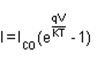Where q is the charge on electron, V is the drop across diode, T is the temperature and K is a constant.

On differentiating w.r.t V, we get,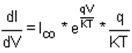The value of (q / KT) at 25°C is approximately 40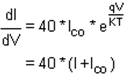Therefore,

or,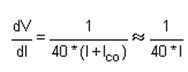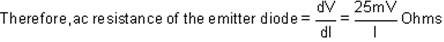To a close approximation the small changes in collector current equal the small changes in emitter current. In the ac equivalent circuit, the current ‘iC' is shown upward because if ‘ie' increases, then ‘iC' also increases in the same direction.

Voltage gain:

Since the ac input voltage source is connected across r'e. Therefore, the ac emitter current is given by

ie = Vin / r'e

or,        Vin = ie r'e

The output voltage is given by Vout = iC (RC || RL)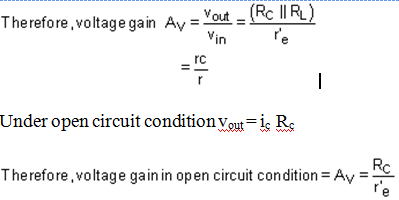Example-1

Find the voltage gain and output of the amplifier shown in fig. 4, if input voltage is 1.5mV.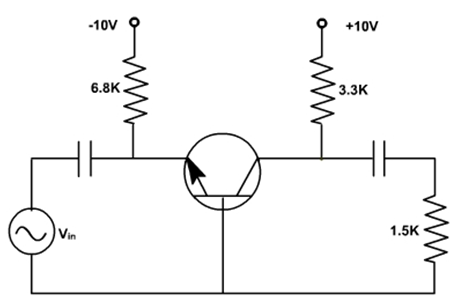Fig. 4

Solution: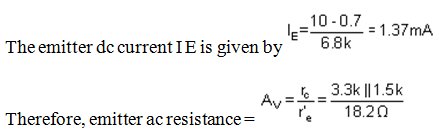or,           AV= 56.6

and, Vout = 1.5 x 56.6 = 84.9 mV

Example-2

Repeat example-1 if ac source has resistance Rs = 100 W .

Solution:

The ac equivalent circuit with ac source resistance is shown in fig. 5.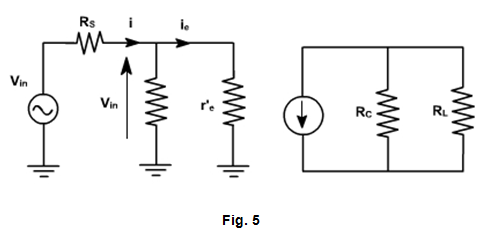The emitter ac current is given by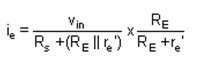or,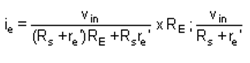Therefore, voltage gain of the amplifier =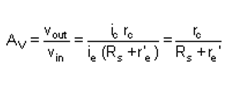and,            Vout = 1.5 x 8.71 =13.1 mV

Small Signal CE Amplifiers:

CE amplifiers are very popular to amplify the small signal ac. After a transistor has been biased with a Q point near the middle of a dc load line, ac source can be coupled to the base. This produces fluctuations in the base current and hence in the collector current of the same shape and frequency. The output will be enlarged sine wave of same frequency.

The amplifier is called linear if it does not change the wave shape of the signal. As long as the input signal is small, the transistor will use only a small part of the load line and the operation will be linear.

On the other hand, if the input signal is too large the fluctuations along the load line will drive the transistor into either saturation or cut off. This clips the peaks of the input and the amplifier is no longer linear.

The CE amplifier configuration is shown in fig. 1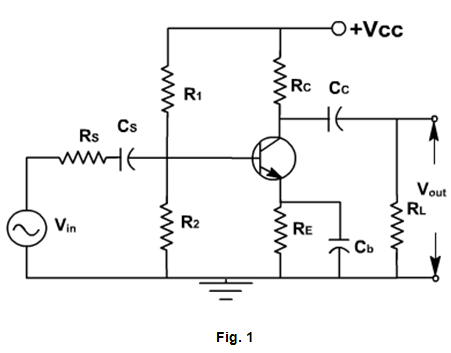The coupling capacitor (CC ) passes an ac signal from one point to another. At the same time it does not allow the dc to pass through it. Hence it is also called blocking capacitor.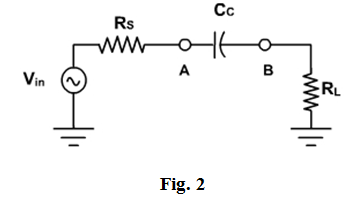Fig. 2

For example in fig. 2, the ac voltage at point A is transmitted to point B. For this series reactance XC should be very small compared to series resistance RS. The circuit to the left of A may be a source and a series resistor or may be the Thevenin equivalent of a complex circuit. Similarly RL may be the load resistance or equivalent resistance of a complex network. The current in the loop is given by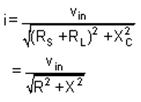As frequency increases,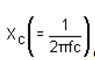decreases, and current increases until it reaches to its maximum value  Vin / R. Therefore the capacitor couples the signal properly from A to B when XC<< R. The size of the coupling capacitor depends upon the lowest frequency to be coupled. Normally, for lowest frequency XC < 0.1R is taken as design rule.

The coupling capacitor acts like a switch, which is open to dc and shorted for ac.

The bypass capacitor Cb is similar to a coupling capacitor, except that it couples an ungrounded point to a grounded point. The Cb capacitor looks like a short to an ac signal and therefore emitter is said ac grounded. A bypass capacitor does not disturb the dc voltage at emitter because it looks open to dc current. As a design rule XCb < 0.1RE  Analysis of CE amplifier:

In a transistor amplifier, the dc source sets up quiescent current and voltages. The ac source then produces fluctuations in these current and voltages. The simplest way to analyze this circuit is to split the analysis in two parts: dc analysis and ac analysis. One can use superposition theorem for analysis .

AC & DC Equivalent Circuits:

For dc equivalent circuit, reduce all ac voltage sources to zero and open all ac current sources and open all capacitors. With this reduced circuit shown in fig. 3 dc current and voltages can be calculated.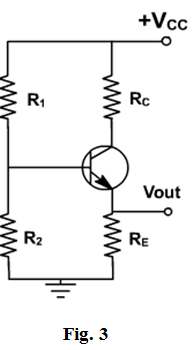For ac equivalent circuits, reduce dc voltage sources to zero and open current sources and short all capacitors. This circuit is used to calculate ac currents and voltage as shown in fig. 4.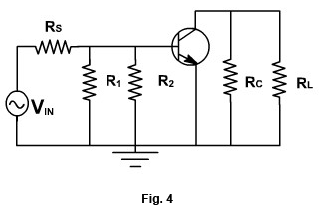The total current in any branch is the sum of dc and ac currents through that branch. The total voltage across any branch is the sum of the dc voltage and ac voltage across that branch.
Phase Inversion: Because of the fluctuation in base current; collector current and collector voltage also swings above and below the quiescent voltage. The ac output voltage is inverted with respect to the ac input voltage, meaning it is 180º out of phase with input.
During the positive half cycle, base current increases causing the collector current to increase. This produces a large voltage drop across the collector resistor; therefore, the voltage output decreases and negative half cycle of output voltage is obtained. Conversely, on the negative half cycle of input voltage less collector current flows and the voltage drop across the collector resistor decreases, hence collector voltage increases. We get the positive half cycle of output voltage as shown in fig. 5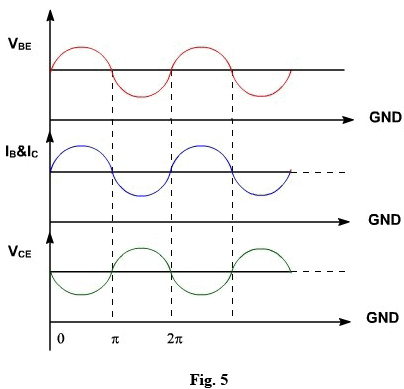Lowest frequency.

Consider the dc equivalent circuit fig. 1.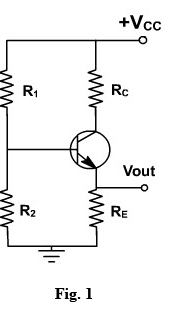Assuming IC = ICE(approx), the output circuit voltage equation can be written as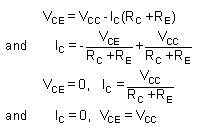The slop of the d.c load line is .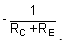When considering the ac equivalent circuit, the output impedance becomes RC || RL which is less than (RC +RE).
In the absence of ac signal, this load line passes through Q point. Therefore ac load line is a line of slope (-1 / (RC || RL) ) passing through Q point. Therefore, the output voltage fluctuations will now be corresponding to ac load line as shown in fig. 2. Under this

condition, Q-point is not in the middle of load line, therefore Q-point is selected slightly upward, means slightly shifted to saturation side.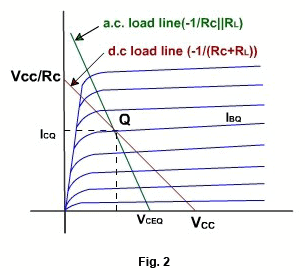Offer running on EduRev: Apply code STAYHOME200 to get INR 200 off on our premium plan EduRev Infinity!

,

,

,

,

,

,

,

,

,

,

,

,

,

,

,

,

,

,

,

,

,

;